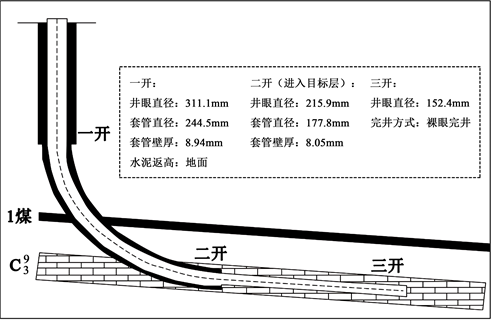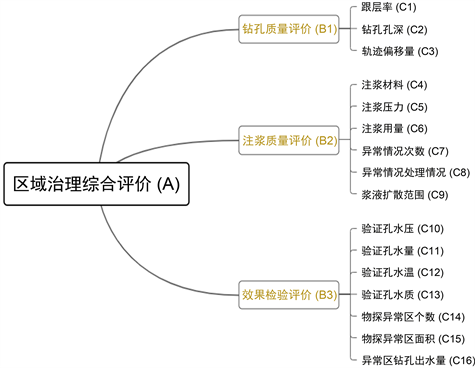﻿ 顾北煤矿13521采区注浆效果治理多因素综合模拟评价

顾北煤矿13521采区注浆效果治理多因素综合模拟评价Comprehensive Simulation Evaluation of the Effect of Slurry Injection in 13521 Mining Area of Gubei Coal Mine

Abstract: In recent years, along with the exploitation of shallow resources in the eastern mining areas, in or-der to meet the needs of resources, coal mining has also developed from shallow mining to deep mining, and the “three highs and one disturbance” brought about by deep coal seam mining is the problem of deep coal seam mining caused by high stress, high temperature, high water pressure and severe mining disturbance. Among them, the problem of sudden water caused by high water pressure is particularly serious. Gubei 13521 work surface due to the increase in mining depth, the bottom Taiyuan group ash karst crack pressure aquifer has a greater impact on the working surface mining, so in order to reduce mining risk, there is urgent need to carry out ground area manage-ment. At this stage, it is more efficient method for regional governance: injection reinforcement. In this paper, taking the regional management project of 13521 working surface slurry in the eastern Gubei coal mine as an example, this paper compares the actual evaluation effect of slurry with the simulation evaluation of AHP, and has some reliability compared with the actual comparison.

1. 引言

13521采区工作面主要受底部太原组灰岩岩溶裂隙水影响，其中C3I组灰岩含水层为1煤层底板直接充水含水层，厚度为29.6~35 m，平均厚度为32.3 m。通过底板的构造裂隙与采动裂隙影响工作面，因此采用注浆加固来进行水害防治。本文以顾北煤矿为例，结合AHP对煤层底板区注浆区域综合治理进行模拟评价 。

2. 注浆孔区域概况

13521工作面主体为向东倾斜的单斜构造 。根据工作面的综合历次三维地震数据、，工作面发育断层14条，其中对采掘影响较大的断层2条，分别为FS854和FS960 (如下表1)。13521工作面煤层发育稳定，属稳定型煤层。Table 1. Three-dimensional seismic exploration and interpretation of faults in 13521 working face

13521工作面地面区域探查治理范围内施工3个钻探主孔、26个钻探分支孔，钻孔钻进及注浆工作于2021年2月27日全部完成。本次探查治理共施工3个钻探主孔，26个钻探分支孔，共完成钻探工程量17,576 m，其结构图如图1所示。Figure 1. Schematic diagram of drilling well structure for exploration and treatment of surface area in Nanyi 1 coal mining area

3. 评价指标的设计Figure 2. Hierarchical analysis structure diagram

3.1. 判断矩阵构造与计算Table 2. Discrimination scale of each element in the judgment matrixTable 3. Judgment matrix and weights for comprehensive evaluation of regional governanceTable 4. Judgment matrix and weights for borehole quality evaluationTable 5. Judgment matrix and weights for grouting quality evaluationTable 6. Judgment matrix and weights of effect test evaluationTable 7. The value table of random consistency index R.I. with different matrix betweennesses

$B=\left[\begin{array}{ccc}{b}_{11}& \cdots & {b}_{1n}\\ ⋮& \ddots & ⋮\\ {b}_{n1}& \cdots & {b}_{nn}\end{array}\right]$

1) 计算矩阵每行所有元素的几何平均数

${W}_{i}=\sqrt[n]{{\prod }_{j=1}^{n}{a}_{ij}},i=1,2,\cdots ,n$，可得， ${W}_{i}={\left({w}_{1},{w}_{2},\cdots ,{w}_{n}\right)}^{\text{T}}$ (3.1)

2) 进行归一化

${W}_{i}=\frac{{w}_{i}}{{\sum }_{j=1}^{n}{w}_{j}},i=1,2,\cdots ,n$，可得， ${W}_{i}={\left({w}_{1},{w}_{2},\cdots ,{w}_{n}\right)}^{\text{T}}$ (3.2)

3) 计算判断矩阵最大特征值 ${\lambda }_{\mathrm{max}}$

${\lambda }_{\mathrm{max}}={\sum }_{i=1}^{n}\frac{{\left(Aw\right)}_{i}}{n{w}_{i}}$，其中 ${\left(Aw\right)}_{i}$ 为向量Aw的第i个元素。 (3.3)

4) 一致性检验

$C.R.=\frac{C.I}{R.I}$ (3.4)

$C.I=\frac{{\lambda }_{\mathrm{max}}-n}{n-1}$ (3.5)

${C}_{i}=\underset{j=1}{\overset{m}{\sum }}{c}_{ij}{b}_{j},\text{\hspace{0.17em}}\text{\hspace{0.17em}}i=1,2,\cdots ,n$Table 9. Priority weight of grouting effect evaluation index

3.2. 计算结果及其评价

1) 钻孔质量评价(B1)

2) 注浆质量评价(B2)

3) 效果检验评价(B3)Table 10. Classification of grouting effect grade level

$V=\underset{i=1}{\overset{16}{\sum }}{V}_{i}=\underset{i=1}{\overset{16}{\sum }}{Z}_{i}×{C}_{i}$ (3.6)

4. 结论

1) 根据实际现场采用物探方法检测注浆效果与模拟效果评价大致一致可以确定AHP分析法在注浆效果评价中是可以实现一定的预测效果。

2) 对于注浆加固改善采区工作面灰岩水害防治取得了很好的效果。注浆效果明显，探查治理效果良好。

3) 利用各因素关系、专家打分、MATLAB计算，通过建立层次分析结构、构造与计算判断矩阵、确定各因素的权重以及建立评价等级等步骤，分步完成对于多因素影响评价模型。根据工作面实际评价效果从而推动模型的优化与分析。

 吴基文, 沈书豪, 翟晓荣. 煤层底板注浆加固效果波速探查与评价[J]. 物探与化探, 2014, 38(6): 1302-1306.

 韩云春. 基于采动效应研究的注浆工作面底板突水危险性评价[D]: [硕士学位论文]. 淮南: 安徽理工大学, 2011.

 许延春, 黄磊, 俞洪庆, 罗亚麒, 李鹏飞, 耿浩博, 等. 基于注浆钻孔数据收集的注浆工作面底板突水危险性评价体系[J]. 煤炭学报, 2020, 45(3): 1150-1159.

 慕松利, 李鹏飞, 耿浩博, 韩久方. 底板注浆效果及突水危险性评价法在赵固二矿的应用[J]. 煤炭工程, 2020, 52(4): 67-72.

 王凯. 甚井微裂隙软岩高压注浆渗流特性及应用研究[D]: [博士学位论文]. 徐州: 中国矿业大学, 2020.

 李亚昊, 朱昌淮, 缪长军, 张海涛, 魏健, 丁永俊, 等. 东部矿区煤层底板区域注浆治理效果评价[J]. 地下水, 2020, 42(5): 58-61+67.

 王润红, 郭联宏, 朱文胜, 郭华. 突水系数法在华晟荣煤矿水上带压开采安全评价中的应用[J]. 煤矿安全, 2012, 43(10): 135-138.

 黄小广. 煤矿安全注浆效果检测方法的评价[J]. 煤矿安全, 2001(10): 38-39.

 王邵辉, 刘占宁, 张朋伟, 贾立锋, 王远声, 张大为. 煤矿生产部门技术工作评价方法研究[J]. 煤炭技术, 2021, 40(6): 217-220.

 谢和平, 高峰, 鞠杨, 高明忠, 张茹, 高亚楠, 等. 深部开采的定量界定与分析[J]. 煤炭学报, 2015, 40(1): 1-10.

 柏建彪, 王襄禹, 贾明魁, 侯朝炯. 深部软岩巷道支护原理及应用[J]. 岩土工程学报, 2008, 30(5): 632-635.

 刘瑜. 复杂开采条件下顶板砂岩突水危险性评价[D]: [硕士学位论文]. 淮南: 安徽理工大学, 2015.

 武强, 王金华, 刘东海, 崔芳鹏, 刘守强. 煤层底板突水评价新型实用方法IV: 基于GIS的AHP型脆弱性指数法应用[J]. 煤炭学报, 2009, 34(2): 233-238.

 代礼永. 刘店矿过断层注浆加固技术研究[J]. 中国西部科技, 2015(8): 17-19, 37.

 徐光亮, 刘旭锋. 煤矿破碎围岩注浆加固技术研究现状分析[J]. 煤矿安全, 2016, 47(1): 174-177.

Top